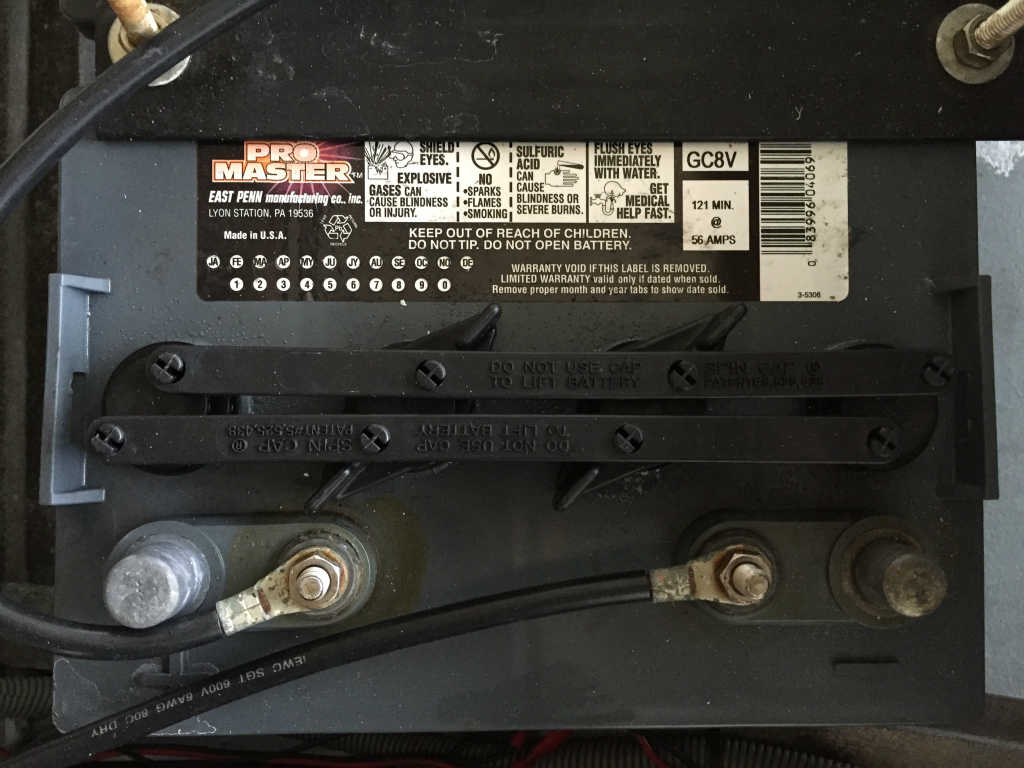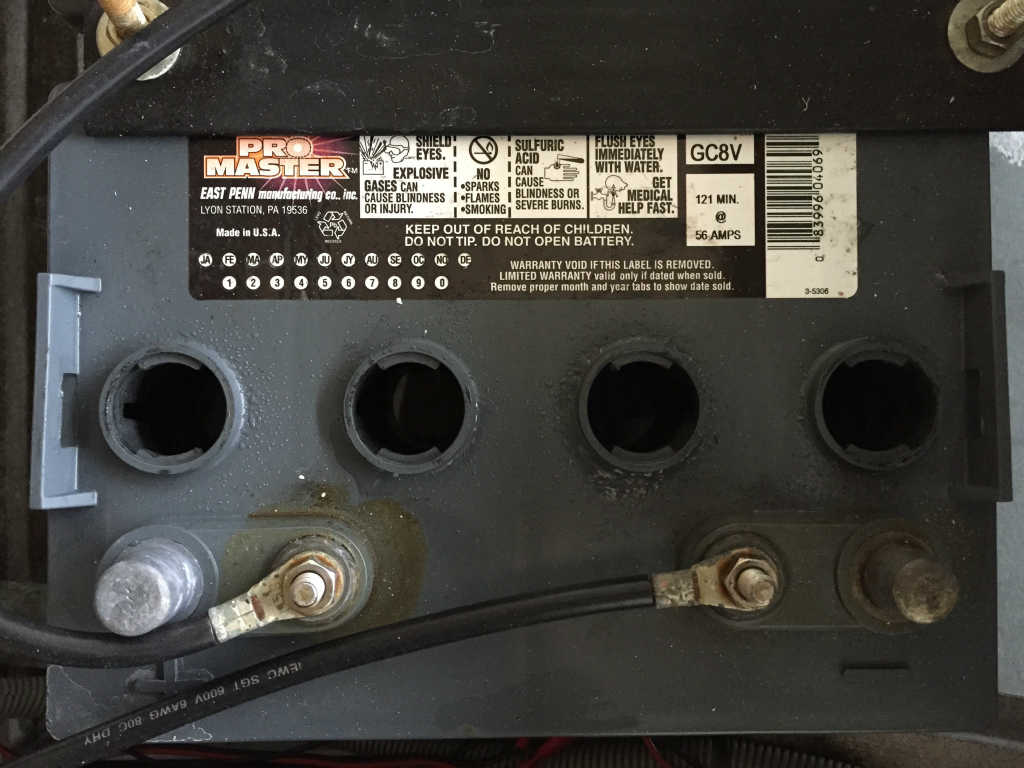# What Voltage Is My Cart?

### Electric Golf Carts:

An electric golf cart will have either four, six or eight golf cart batteries and will have either a 36 volt or 48 volt electrical drive system.

### How to Determine Golf Cart Voltage (36V or 48V):

1)  Lift up your golf cart seat to locate the battery compartment.

2)  Count the number of holes

• Usually 3, 4 or 6 holes

3)  Multiply the number of holes by 2 to determine the golf cart battery voltage

• Each hole/cell is equal to 2 volts

4)  Multiply the golf cart battery voltage by the total number of golf cart batteries

### 36 Volt Golf Cart Example (6V Batteries):

3 holes X 2 volts = 6 volt battery

6 volt battery X 6 total batteries = 36 volt system

### 48 Volt Golf Cart Example (8V Batteries):

4 holes X 2 volts = 8 volt battery

8 volt battery X 6 total batteries = 48 volt system### 48 Volt Golf Cart Example (12V Batteries):

6 holes X 2 volts = 12 volt battery

12 volt battery X 4 total batteries = 48 volt system